# Voltage divider

The voltage divider is a passive linear circuit that is used to create an output voltage that is a fraction of the input voltage. Voltage dividers commonly consist of two resistors. One of the resistors is connected from the input to the output and the second one is connected from the output to ground. You can also use other components like capacitors and inductors. You can find more information about these here:
Capacitive voltage divider
Inductive voltage divider

### Formulas

The output voltage can be calculated with the following formula: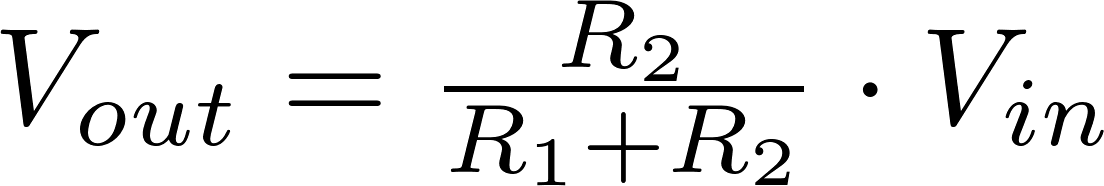The formulas for the other variables can be found using algebra: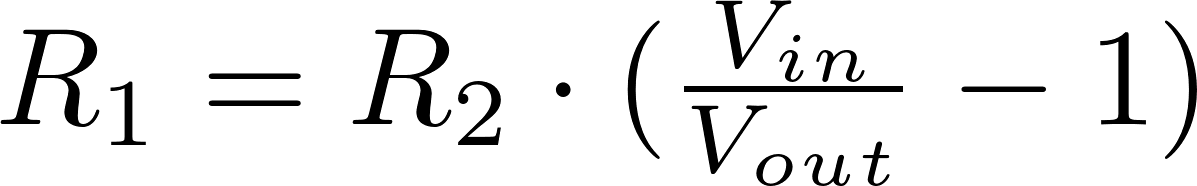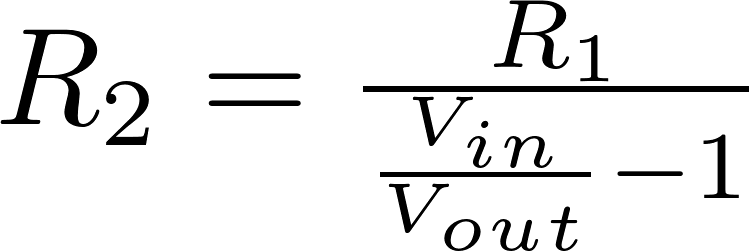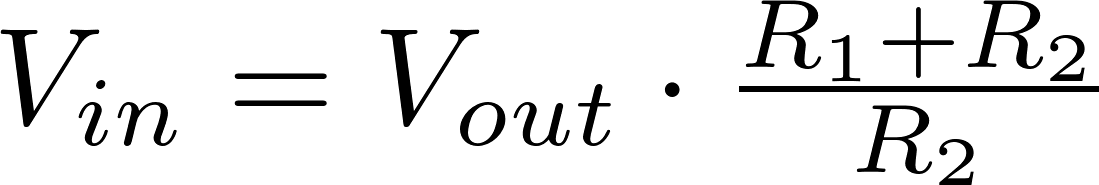V in is the input voltage and is measured in volt (V)
R is the symbol for resistance and is measured in ohm (Ω).
V out is the output voltage and is measured in volt (V)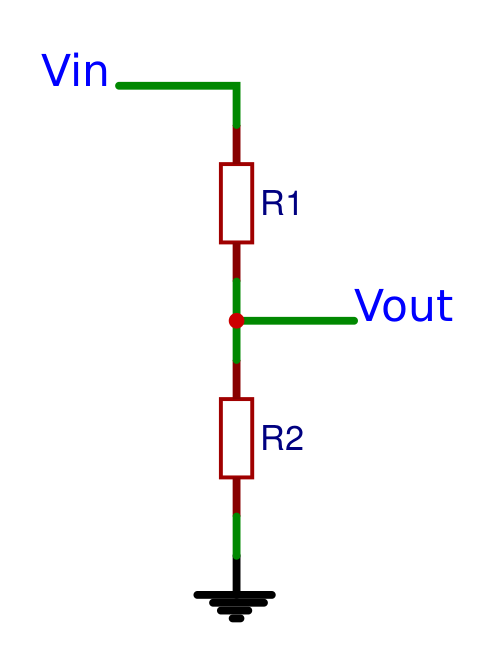### Calculator

Enter three values to calculate the remaining one.